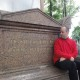# Moscow Mathematical Papyrus

One of the most important objects kept in the Pushkin Museum of Fine Arts is the Moscow Mathematical Papyrus. This papyrus was purchased by Vladimir Golenishchev in 1893 from one of the brothers who found the Pharaoh’s tomb in Deir al-Bahri. The text is written in hieratic and it’s supposed to be compiled around 1850 BC. It measures 5 meters long and 8 cm wide and containing 25 mathematical problems. Unfortunately, not all the problems are readable so we only have information about some of its sections. The 25 problems are:

3. A stick measures 1/3 and 1/5 [=1/3 + 1/5] of 30 = 16.
4. Area of a triangle: 1/2 of 4 x 10 = 20.
5. Pesus of loaves and bread.
6. Rectangle of area = 12 and base = 1/2 and 1/4 of the height. Find the base and the height.
7. Triangle of area = 20 and height = 2 and 1/2 of base. Find the base and the height.
8. Pesus of loaves and bread.
9. Pesus of loaves and bread.
10. Area of a curved surface of a cylinder or a hemisphere.
12. Pesus of beer.
13. Pesus of loaves and beer.
14. Volume of a truncated pyramid.
15. Pesu of beer.
16. Pesu of beer.
17. Triangle of area = 20 and base = 1/3 and 1/15 of height. Find the base and the height.
18. Measuring cloth in cubits and palms.
19. Solve the equation (1 and 1/2)x + 4 = 10.
20. Pesu of 1.000 loaves. Oudjat.
22. Pesus of loaves and beer.
23. Computing the work of a cobbler.
24. Exchange of loaves and beer.
25. Solve the equation 2x + x = 9.

The section of the papyrus which is exhibited in the Pushkin Museum is problem number 14. We can see clearly a figure similar to a trapezium that represents a truncated pyramid and the scribe computed its volume through the formula: V = (h/3) · (a2 + ab + b2). The exact text is:

It is said to thee, a truncated pyramid of 6 ellen in height.

Of 4 ellen of the base, by 2 of the top.

Reckon thou with this 4, squaring. Result 16.

Double thou this 4. Result 8.

Reckon thou with this 2, squaring. Result 4.

Add together this 16, with this 8, and with this 4. Result 28.

Calculate thou 1/3 of 6. Result 2.

Calculate thou with 28 twice. Result 56.

Lo! It is 56! Thou has found rightly.

Location: Pushkin Museum of Fine Arts in Moscow (map)

### 5 responses

1.Is it possible to reproduce this photo on a website. We will make no profit from it, I just can’t find any decent images of the papyrus anywhere.

1.3.